# 2. (8 points) Let {fn}n>ı be a sequence of functions that are defined on R by...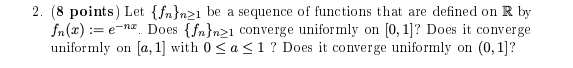2. (8 points) Let {fn}n>ı be a sequence of functions that are defined on R by fn(x):= e-nx. Does {{n}n>1 converge uniformly on [0, 1]? Does it converge uniformly on (a, 1) with 0 <a<1? Does it converge uniformly on (0, 1)?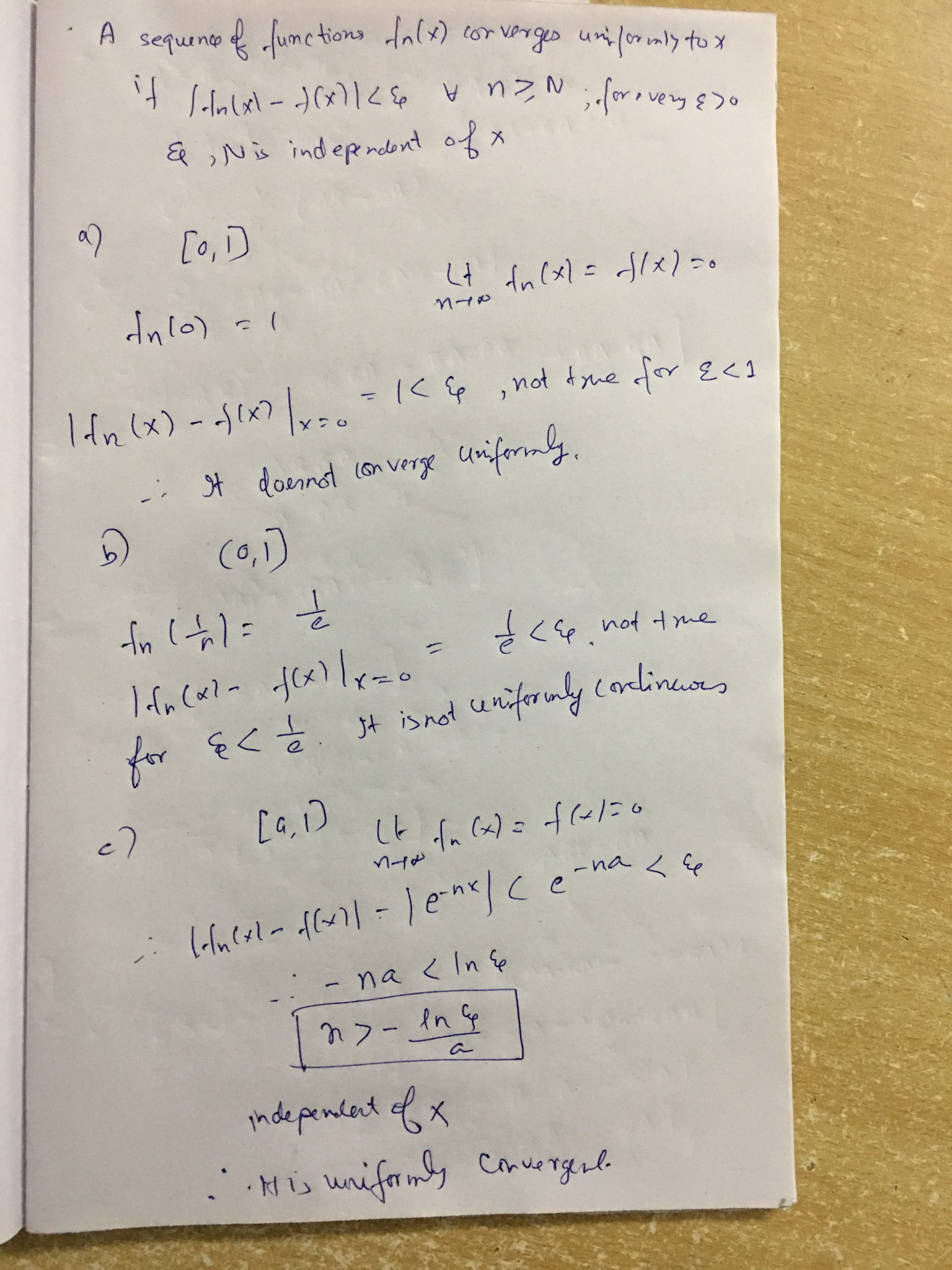#### Earn Coin

Coins can be redeemed for fabulous gifts.

Similar Homework Help Questions
• ### Proposition 7.27. Suppose fn: G + C is continuous, for n > 1, (fn) converges uniformly...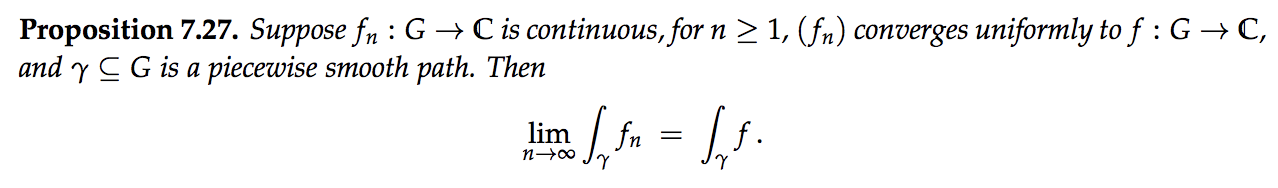Proposition 7.27. Suppose fn: G + C is continuous, for n > 1, (fn) converges uniformly to f :G+C, and y C G is a piecewise smooth path. Then lim n-00 \$. fn = \$. . 7.23. Let fn(x) = n2x e-nx. (a) Show that limn400 fn(x) = 0 for all x > 0. (Hint: Treat x = O as a special case; for x > 0 you can use L'Hôspital's rule (Theorem A.11) — but remember that n is...

• ### 1. (a) Let {fn}neN : [0,00) + R be a sequence of function define by: sin(nx)...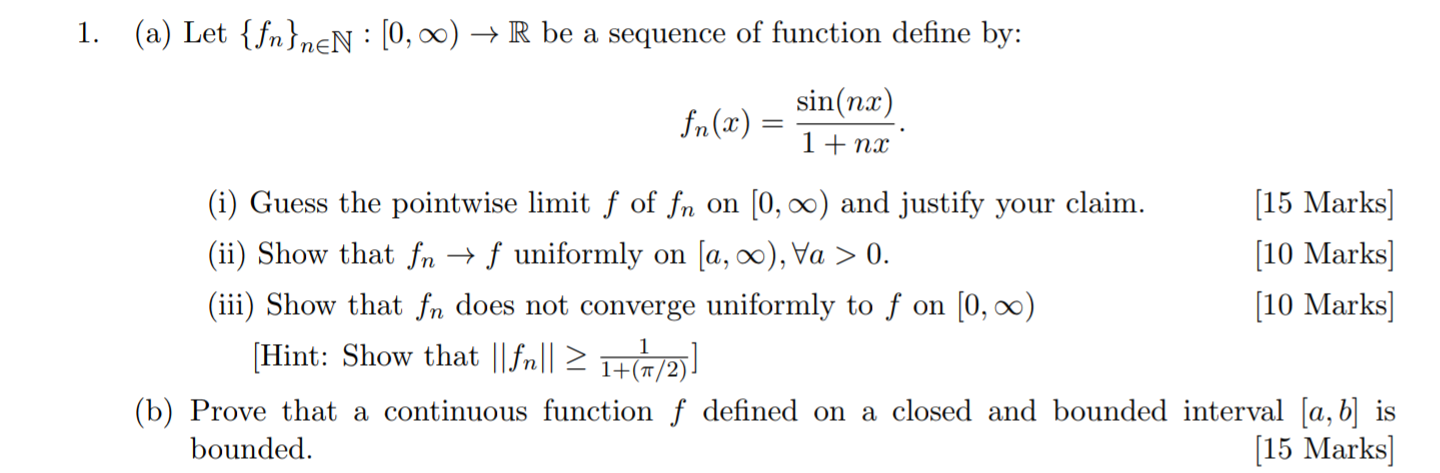1. (a) Let {fn}neN : [0,00) + R be a sequence of function define by: sin(nx) fn(x) 1+ nx (i) Guess the pointwise limit f of fn on (0,00) and justify your claim. [15 Marks] (ii) Show that fn + f uniformly on ſa, 00), Va > 0. [10 Marks) (iii) Show that fn does not converge uniformly to f on (0,00) [10 Marks] (Hint: Show that ||fr|| 21+(1/2) (b) Prove that a continuous function f defined on a closed...

• ### 2. Let a be a positive real number, let r be a real number satisfying r...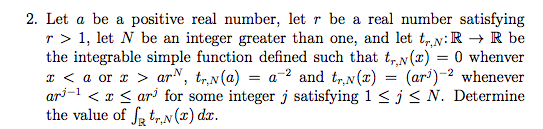2. Let a be a positive real number, let r be a real number satisfying r >1, let N be an integer greater than one, and let tR -R be the integrable simple function defined such that tr,N(r) = 0 whenver x < a or z > ar*, tr,N(a) = a-2 and tr,N(z) = (ar)-2 whenever arj-ıく < ar] for some integer j satisfying 1 < j < N. Determine the value of JR trN(x) dz.

• ### Please prove this, thanks! 2. Let {xn n21 be a sequence in R such that all...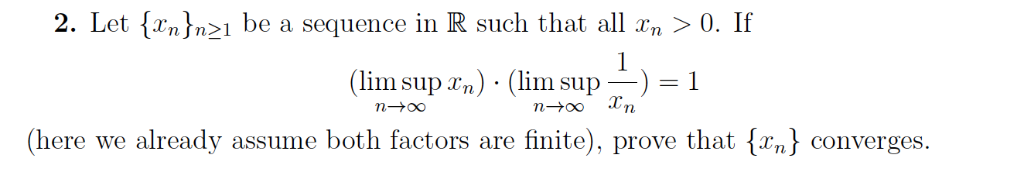Please prove this, thanks! 2. Let {xn n21 be a sequence in R such that all n > 0. If ( lim supra) . (lim supー) = 1 Tn (here we already assume both factors are finite), prove that converges.

• ### 2. Let Xn, n > 1, be a sequence of independent r.v., and Øn (t) =...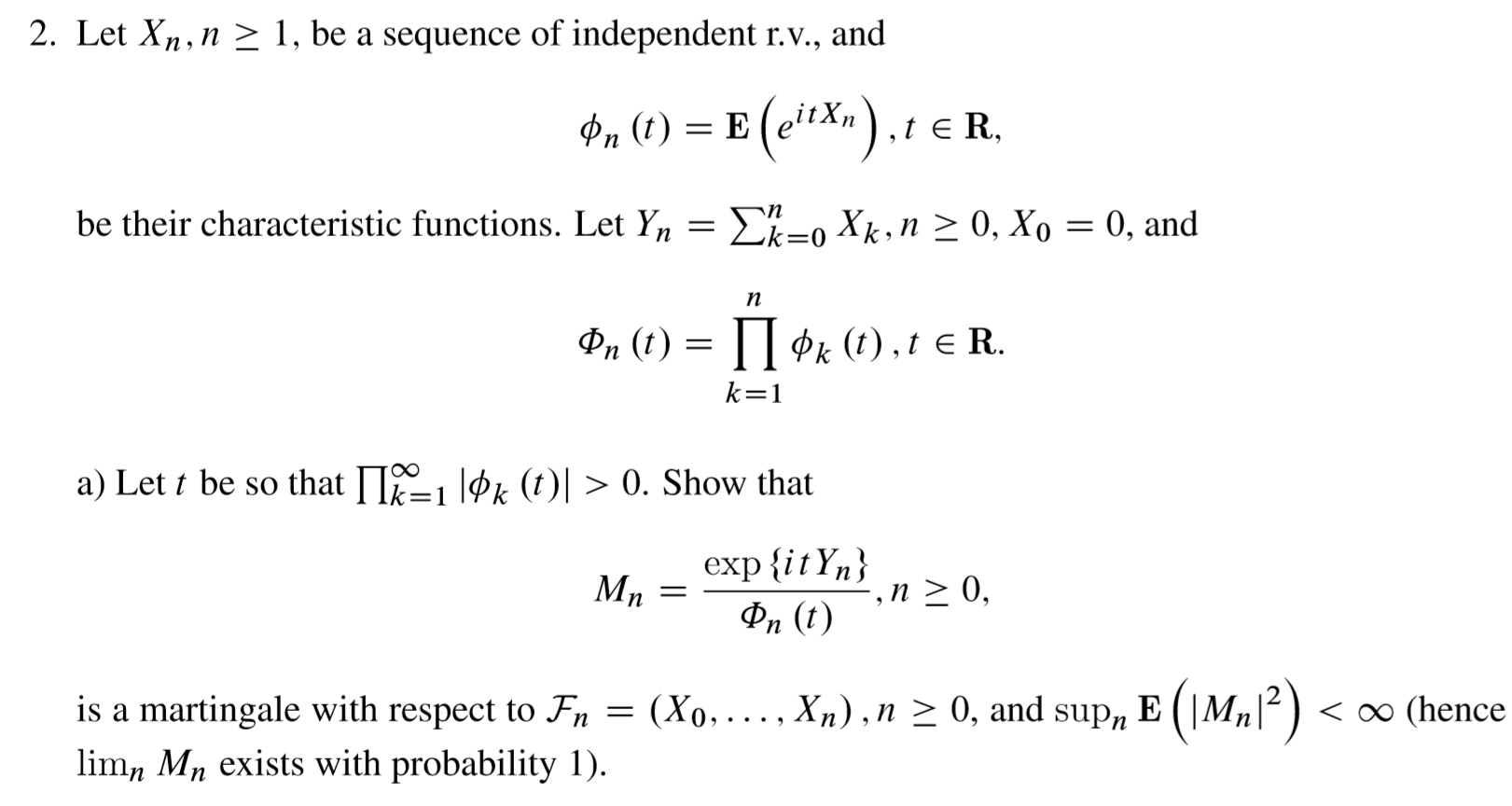2. Let Xn, n > 1, be a sequence of independent r.v., and Øn (t) = E (eitX»), ER be their characteristic functions. Let Yn = {k=0 Xk, n > 0, X0 = 0, and 8. () = {1*: (),ER. k = 1 a) Let t be so that I1=1 løk (t)) > 0. Show that _exp{itYn} ?, n > 0, On (t) is a martingale with respect to Fn = (Xo, ...,Xn), n > 0, and sup, E (M,|2)...

• ### Many thanks!! (a) Let fn(x) max(1 - |x -n|,0) for each n 2 1. Show that...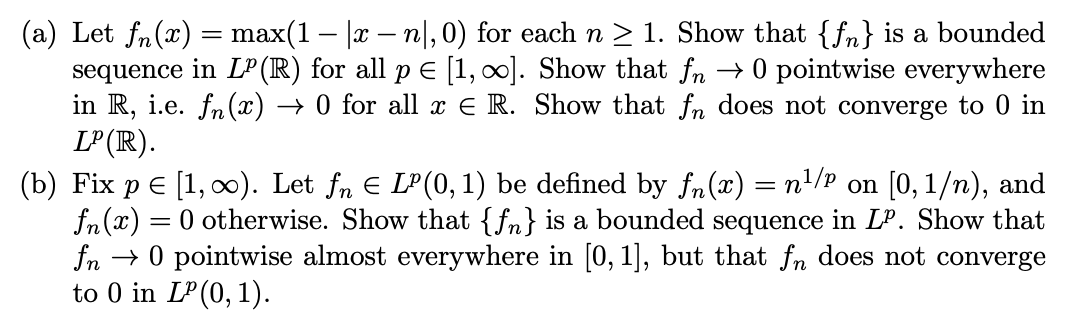Many thanks!! (a) Let fn(x) max(1 - |x -n|,0) for each n 2 1. Show that {fn} is a bounded sequence in LP (R) for all p E [1, 00]. Show that fn >0 pointwise everywhere in R, i.e. fn(x) -> 0 for all x E R. Show that fn does not converge to 0 in LP (R) (b) Fix p E 1, o0). Let fn E LP(0, 1) be defined by fn(x) n1/? on [0,1/n), and fn(x)0 otherwise. Show...

• ### (Exercise 9.2) Let f,, : R → R, fn(x)-n and f : R → R, f(x) fn does not converge uniformly to f (i.e. fn /t f uniformly...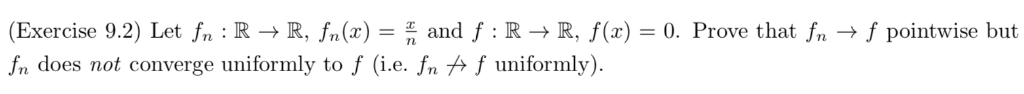(Exercise 9.2) Let f,, : R → R, fn(x)-n and f : R → R, f(x) fn does not converge uniformly to f (i.e. fn /t f uniformly) 0. Prove that fn → f pointwise but (Exercise 9.2) Let f,, : R → R, fn(x)-n and f : R → R, f(x) fn does not converge uniformly to f (i.e. fn /t f uniformly) 0. Prove that fn → f pointwise but

• ### Please answer it step by step and Question 2. uniformly converge is defined by *f=0* clear...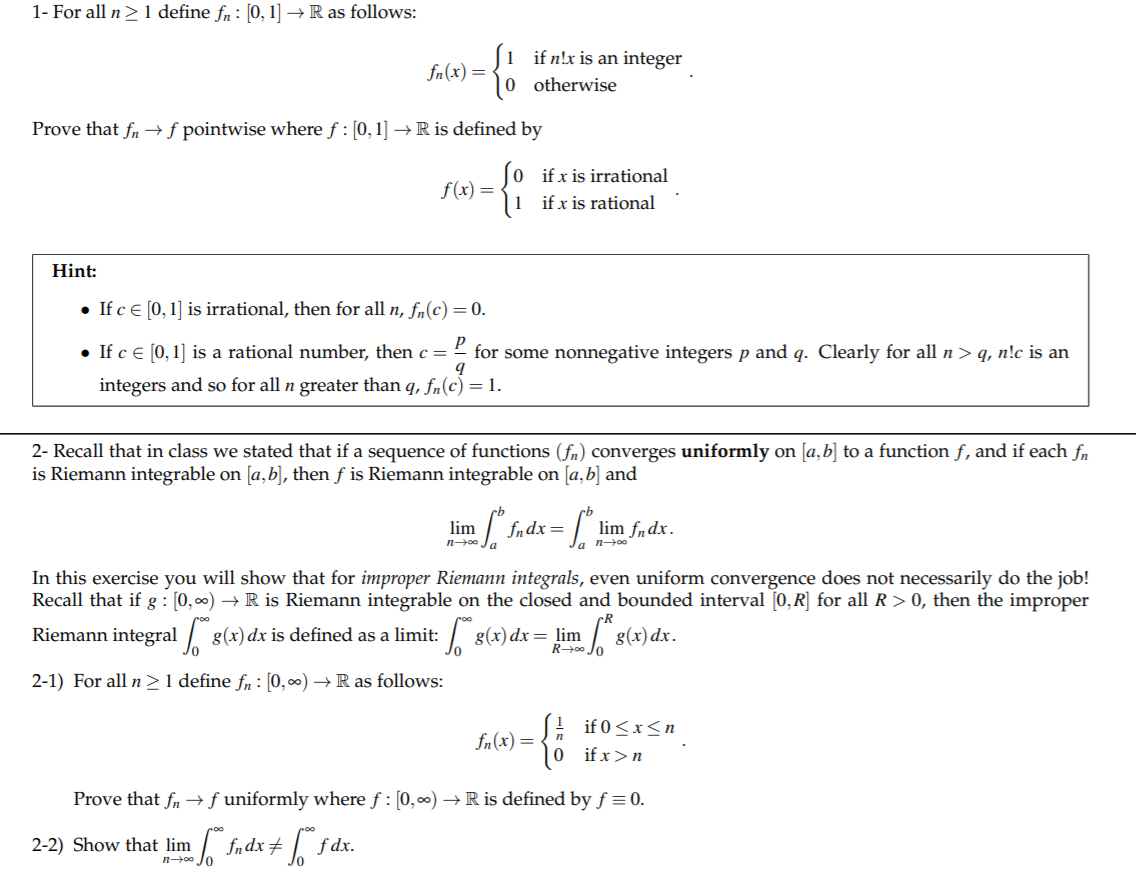Please answer it step by step and Question 2. uniformly converge is defined by *f=0* clear handwritten, please, also, beware that for the x you have 2 conditions , such as x>n and 0<=x<=n 1- For all n > 1 define fn: [0, 1] → R as follows: (i if n!x is an integer 10 otherwise Prove that fn + f pointwise where f:[0,1] → R is defined by ſo if x is irrational f(x) = 3 11 if x...

• ### (10) Suppose that fn : R -> R is a sequence of functions such that for every xo E R there exist a neighborhood N(ro)...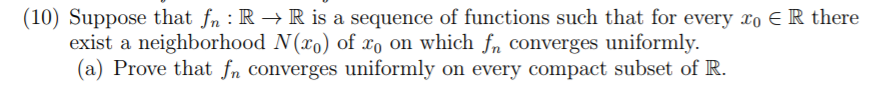(10) Suppose that fn : R -> R is a sequence of functions such that for every xo E R there exist a neighborhood N(ro) of xo on which fn converges uniformly. (a) Prove that fn converges uniformly on every compact subset of R (10) Suppose that fn : R -> R is a sequence of functions such that for every xo E R there exist a neighborhood N(ro) of xo on which fn converges uniformly. (a) Prove that fn...

• ### (3) Prove that the sequence fn (x(max10,z - n))2 does not converge uniformly on IR, but converges...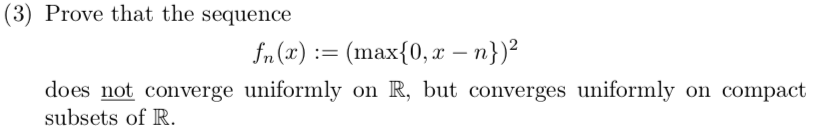(3) Prove that the sequence fn (x(max10,z - n))2 does not converge uniformly on IR, but converges uniformly on compact subsets of R (3) Prove that the sequence fn (x(max10,z - n))2 does not converge uniformly on IR, but converges uniformly on compact subsets of R

Free Homework App